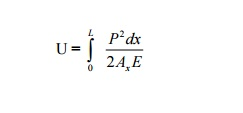Home | | Strength of Materials | | Strength of Materials II | Energy Principles: Strain Energy, Proof Stress

# Energy Principles: Strain Energy, Proof Stress

Whenever a body is strained, the energy is absorbed in the body. The energy which is absorbed in the body due to straining effect is known as strain energy. The stress induced in an elastic body when it possesses maximum strain energy is termed as its proof stress.

STRAIN ENERGY

Whenever a body is strained, the energy is absorbed in the body. The energy which is  absorbed in the body due to straining effect is known as strain energy. The strain energy stored in the  body is equal to the work done by the applied load in stretching the body.

PROOF STRESS

The stress induced in an elastic body when it possesses maximum strain energy is termed as  its proof stress.

Derive the expression for strain energy in Linear Elastic Systems for the following cases. (i)  Axial loading (ii) Flexural Loading (moment (or) couple)

Let us consider a straight bar of Length L, having uniform cross- sectional area A. If an axial  load P is applied gradually, and one,ifstoredthe bar  as strain energy (U) in the body, will be equal to average force (1/2 P) multiplied by the  deformation ?.

Thus  U   =   �   P.   ?

But   ?   =   PL   /   AE

U = � P. PL/AE  = P2 L / 2AE                                           ---------- (i)

If, however the bar has variable area of cross section, consider a small of length dx and area  of cross section Ax. The strain energy dU stored in this small element of length dx will be,  from equation (i)The total strain energy U can be obtained by integrating the above expression over the length of the bar.Let us now consider a member of length L subjected to uniform bending moment M.  Consider an element of length dx and let di be the change in the slope of the element due to  applied moment M. If M is applied gradually, the strain energy stored in the small element will beLet us now consider a member of length L subjected to uniform bending moment M.  Consider an element of length dx and let di be the change in the slope of the element due to  applied moment M. If M is applied gradually, the strain energy stored in the small element will beStudy Material, Lecturing Notes, Assignment, Reference, Wiki description explanation, brief detail
Civil - Strength of Materials - Energy Principles : Energy Principles: Strain Energy, Proof Stress |

Related Topics